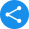# NCERT Class 7 Maths Book PDFView Notes

NCERT books are an integral part of the curriculum of any CBSE school. The students prefer these books because they find these easier to study owing to its simplicity. PDF version of these texts and its related materials are also readily available. NCERT books for class 7 maths, is no exception.

Students can download the PDF and access it from anywhere. They can use the solutions to class 7th NCERT maths book and understand the concepts better. NCERT maths class 7 textbook is ideal for young students because these books would enable them to understand each of the topics comprehensively.

These NCERT Solutions help students in learning the concept in a comprehensive manner and allow them to score better marks in their examination.

### NCERT Solutions for Class 7

1. How many chapters are there in the NCERT Books for Class 7 Maths?

Ans: There are a total of 15 chapters in the NCERT Books for Class 7 Maths. Below are the names of the chapters:

• Chapter 1 - Integers

• Chapter 2 - Fractions and Decimals

• Chapter 3 - Data Handling

• Chapter 4 - Simple Equations

• Chapter 5 - Lines and Angles

• Chapter 6 - Lines and Angles

• Chapter 7 - The Triangle and Its Properties

• Chapter 8 - Congruence of Triangles

• Chapter 9 - Comparing Quantities

• Chapter 10 - Rational Numbers

• Chapter 11 - Practical Geometry

• Chapter 12 - Perimeter and Area

• Chapter 13 - Algebraic Expression

• Chapter 14 - Exponents and Power

• Chapter 15 - Symmetry

• Chapter 16 - Visualising Solid Shape

2. What is the sixth chapter of Class 7 Maths NCERT textbook all about?

Ans: The sixth chapter of Class 7 Maths NCERT textbook depicts The Triangle and its Properties. After Chapter 5 Lines and Angles, this is the second chapter related to Geometry in NCERT 7th maths textbook. This chapter is all about triangles.

It includes an in-depth discussion regarding various types of triangles. There is an elaborate discussion on the properties of triangles, which enable students to have a clear concept of this topic. In this chapter, the students will also learn the concepts of altitude of triangles and exterior angles. Moreover, students will get an introduction of the two specialised sub-types of triangles: equilateral and isosceles. They will also learn about the Pythagorean Theory, which will be helpful in their higher studies too.

3. What are the benefits of referring to the Class 7 Maths NCERT textbook?

Ans: Maths is a very important subject. To hold strong command over the fundamental concepts of each topic, every student should practise the problems of Mathematics very well. They can also take help from the NCERT books for class 7 Maths and the solutions of it which are available online. These books introduce a Class 7 student to some critical concepts like that of Arithmetic, Algebra, and Geometry.

NCERT books are an integral part of the curriculum of any CBSE school. The students prefer these books because they find them easier to study owing to its simplicity. NCERT books for class 7 maths is no exception. NCERT maths class 7 textbook is ideal for young students because these books would enable them to understand each of the topics comprehensively.

4. How can I download the NCERT Books for Class 7 Maths?

Ans: Students can download the PDF of NCERT Books for Class 7 Maths and access it from anywhere, be it the official website of NCERT or the official site of Vedantu. One can also download it from Vedantu app as well. In that case, they have to download the app on their phone or any smart device beforehand. These NCERT books are accessible for absolutely free of cost.

Share this with your friendsShare